Next: 3.5 Strain and Bulk Up: 3. Strain and Semiconductor Previous: 3.3 Stress-Strain Relation

# 3.4 Miller Index Notation

The position of a crystal plane is determined by three independent non-collinear points. If these points reside on independent crystal axes, the plane can be characterized in units of the axes. However, it is more convenient to describe the planes location via Miller indices [164,162]. The Miller indices are a triplet of integer values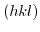, which denote the ratio between the points that intercept the plane and the chosen crystal axes. The Miller indices can be found as follows:

• Define three lattice vectors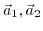, and. In the case of a cubic crystal, the lattice vectors are chosen along the edges of the crystallographic unit cell (unit cube).
• Identify the intercepting points, andbetween the plane and the lattice vectors, and express them in units of the lattice vectors, and.
• Calculate the reciprocal of the, andand choose the smallest three integer valuesthat have a greatest common divisor of one.

Negative indices are denoted by a bar above their value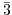or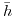. If there is no interception between an axis and the plane, the Miller index is 0 (they intercept in infinity). Depending on the brackets used, their meaning can be further distinguished:

•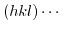round brackets denote a certain plane or the vector perpendicular to the plane
•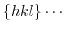curly braces stand for all planes that are equivalent todue to the symmetry of the crystal.
•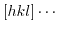these brackets mean a given direction in the crystall.
•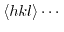angle brackets describe all directions that are equivalent to the direction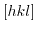.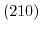means that the plane intersects the axis vectors at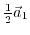and at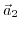. Additionally, the direction vectoris always perpendicular to the plane, for cubic crystal structures.Next: 3.5 Strain and Bulk Up: 3. Strain and Semiconductor Previous: 3.3 Stress-Strain Relation

T. Windbacher: Engineering Gate Stacks for Field-Effect Transistors